Next: Buckling analysis Up: Types of analysis Previous: Frequency analysis   Contents

### Complex frequency analysis

This procedure is used to calculate the eigenvalues and eigenmodes taking the Coriolis forces into account. The latter forces apply as soon as one performs calculations in a rotating frame of reference. Therefore, using the *DLOAD card to define a centrifugal speed in a *FREQUENCY step automatically triggers Coriolis forces. However, in a lot of applications the Coriolis forces are quite small and can be neglected. They may be important for very flexible rotating structures such as thin disks mounted on long rotating axes (rotor dynamics).

The presence of Coriolis forces changes the governing equation into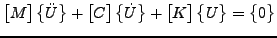(303)

In a *FREQUENCY analysis the term with the Coriolis matrixis lacking. Now, the solution to the above equation is assumed to be a linear combination of the eigenmodes without Coriolis: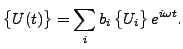(304)

Substituting this assumption into the governing equation and premultiplying the equation with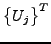leads to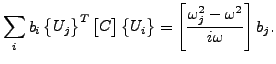(305)

Writing this equation for each value of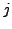yields an eigenvalue problem of the form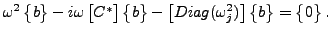(306)

This is a nonlinear eigenvalue problem which can be solved by a Newton-Raphson procedure. Starting values for the procedure are the eigenvalues of the *FREQUENCY step and some values in between. In rare cases an eigenvalue is missed (most often the last eigenvalue requested).

One can prove that the eigenvalues are real, the eigenmodes, however, are usually complex. Therefore, instead of requesting U underneath the *NODE FILE card yielding the real and imaginary part of the displacements it is rather instructive to request PU leading to the size and phase. With the latter information the mode can be properly visualized in CalculiX GraphiX.

Finally, notice that no *DLOAD card of type CORIO is needed in CalculiX. A loading of type CENTRIF in a preceding *STATIC step is sufficient. The usual procedure is indeed:

1. a *STATIC step to define the centrifugal force and calculate the deformation and stresses (may contain NLGEOM, but does not have to).
2. a *FREQUENCY step with PERTURBATION to calculate the eigenfrequencies and eigenmodes taking the centrifugal forces, stress stiffness and deformation stiffness into account. The *FREQUENCY card must include the parameter STORAGE=YES.
3. a *COMPLEX FREQUENCY,CORIOLIS step to include the Coriolis forces.Next: Buckling analysis Up: Types of analysis Previous: Frequency analysis   Contents
guido dhondt 2018-12-15